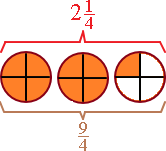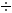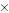Improper Fractions and Mixed Numbers

Improper Fractions and Mixed Numbers

An improper fraction can be written as a mixed number.
The diagram below shows that a mixed number and an improper fraction can both represent the same amount.To change an improper fraction to a mixed number, divide the numerator by the denominator. The fraction bar stands for "divided by", then  means "nine divided by four", then

94 = 2 with 1 as a remnant.

The 2 is the number of wholes and 1 is the number of fourths, so 9/4 is equal to 2 1/4.

To write a mixed number as an improper fraction, just multiply and add. Lets take 2 1/4: first multiply the denominator(4) of the fraction part by the whole-number part(2).

24 = 8

then add the numerator part of the fraction(1) to this amount

8 + 1 = 9

this becomes the new numerator of the improper fraction. The denominator remains the same, so

2 1/4

Try it yourself

Complete each table.
1.
 Division Improper Fraction Mixed Number 163 16/3
2.
 Division Improper Fraction Mixed Number 192 9 1/2
3.
 Division Improper Fraction Mixed Number 11/9

4.

 Division Improper Fraction Mixed Number 28/7

5.

 Division Improper Fraction Mixed Number 4 4/5

1.

 Division Improper Fraction Mixed Number 5 1/3
2.
 Division Improper Fraction Mixed Number 19/2

3.

 Division Improper Fraction Mixed Number 119 1 2/9

4.

 Division Improper Fraction Mixed Number 287 4

5.

 Division Improper Fraction Mixed Number 245 24/5